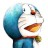# 杰拉斯的博客

## [ACM]简单回溯——猜牌游戏杰拉斯 | 时间：2012-06-02, Sat | 6,619 views

### 猜牌游戏

```100 3
100 5
23205 3
```

#### 【输出样例】

```1 2 50
1 4 25
1 5 20
2 5 10
LIE
3 85 91
5 51 91
7 39 85
7 51 65
13 21 85
13 35 51
15 17 91
17 21 65
17 35 39
```

```#include<stdio.h>
//n 已找到的因子数, q 当前因子, a 已找到的因子, num 情况数
void Solve(int s, int k, int n, int q, int a[], int &num){
if(q > 100){	//若因子超出100，则结束
return;
}
if(n > k){	//若因子数量足够
if(s == 1){	//若s完全除尽
for(int i = 1; i <= k; ++i){	//输出所有因子
printf("%d ", a[i]);
}
printf("\n");
++num;
}
return;
}
if(s % q == 0){	//若q是s的因子
a[n] = q;	//存入数组
Solve(s / q, k, n + 1, q + 1, a, num);	//下层递归
}
Solve(s, k, n, q + 1, a, num);	//寻找下一个因子
}

int main(){
int s, k;
while(~scanf("%d%d", &s, &k)){
int num = 0, a = {0};
Solve(s, k, 1, 1, a, num);
if(!num)	//若情况总数为0
printf("LIE\n");	//说明没找到
}
return 0;
}
```